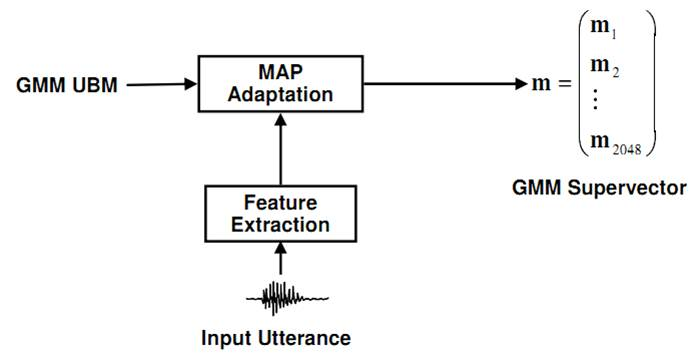## 1.Gaussian Mixture Models(GMM)

For a D-dimensional feature vector, x, the mixture density used for the likelihood function is deﬁned as

$p(x|\lambda) = \sum_{i=1}^{M}w_{i}p_{i}(x)$.

The density is a weighted linear combination of $M$ unimodal Gaussian densities , $p_{i}(x)$
$p_{i}(x) = \frac{1}{(2\pi)^{D/2}|\sum _{i}|^{1/2}} exp[-\frac{1}{2}(x-\mu_{i})^{T}(\sum _{i})^{-1}(x-\mu_{i})]$.

## 2.Support Vector Machines(SVM)

An SVM is a two-class classifier constructed from sums of a kernel function $K(• , •)$

$f(x) = \sum_{i=1}{N}\alpha_{i} t_{i} K(x,x_{i}) + d$.

$t_{i}$ are the ideal outputs, $\sum_{i=1}{N}\alpha_{i} t_{i}=0$ and $\alpha_{i} > 0$,$x_{i}$ are support vectors and obtained from the training set by an optimization process.
$K(. , .)$ is constrained to have certain properties (the Mercer condition), so that it can be expressed as
$K(x,y) = b(x)^{T} b(y)$.

Kernel function examples: http://www.shamoxia.com/html/y2010/2292.html

## 3.GMM-SVM

GMM Supervectors：given a speaker utterance, GMM-UBM training is performed by MAP adaptation of the means $m_{i}$, and we form a GMM supervector $m = [m_{i}]$GMM Supervectors Linear Kernel: a natural distance between the two utterances is the KL divergence, while it does n't satisfy the Mercer condition, we consider the following approximation

\begin{align} D(g_{a}\parallel g_{b}) = &\int_{R^{n}}g_{a}xlog\left(\frac{g_{a}(x)}{g_{b}(x)} \right)dx \\ \leq & \sum_{i=1}^{N} \lambda _{i}D(N(.;m_{i}^{a},\Sigma _{i})\parallel N(.;m_{i}^{b},\Sigma _{i}))\\ = &\frac{1}{2}\sum_{i=1}^{N}\lambda_{i}(m_{i}^{a}-m_{i}^{b})\Sigma ^{-1}(m_{i}^{a}-m_{i}^{b}) \end{align}

we can define a new distance formula,
$d(m^{a},m^{b}) = \frac{1}{2} \sum_{i=1}^{N}\lambda_{i}(m_{i}^{a}-m_{i}^{b})\Sigma_{i}^{-1}(m_{i}^{a}-m_{i}^{b})$

From the distance, we can find the corresponding inner product which is the kernel function.

## 4.SVM-NAP

Nuisance Attribute Projection(NAP) attempts to remove the unwanted within-class variation of the observed feature vectors. This is achieved by applying the transform

$y' = P_{n}y = (I-V_{n}V_{n}^{T})y$.

where Vn is the principal directions of within-class variability.

The SVM NAP constructs a new kernel,

\begin{align} K(m^{a},m^{b}) =& [Pb(m^{a})]^{T}[Pb(m^{b})]\\ =& b(m^{a})^{t}Pb(m^{b})\\ =& b(m^{a})^{t}(I-vv^{t})b(m^{b}) \end{align}.

where $P$ is a projection ($P^{2} = P$) , $v$ is the direction being removed from the SVM expansion space. $b(.)$ is the SVM expansion, and $\parallel v\parallel ^{2} = 1$.

## 5.Experiments on NIST SRE 2010

In the experiments, I use the spro4 to extract MFCC and LPCC features, and use Alize to build GMM-UBM and all of the remained tasks.

## References

Douglas A. Reynolds, T. F. Quatieri, and R. Dunn, Speaker verication using adapted Gaussian mixture models, Digital Signal Processing, vol. 10, no. 1-3, pp. 1941, 2000.
W. Campbell, “Generalized linear discriminant sequence kernels for speaker recognition,” in IEEE International Conference on Acoustics, Speech, and Signal Processing, vol. 1, 2002, pp. 161–164.
W. M. Campbell, D. E. Sturim, D. A. Reynolds, A. Solomonoff, SVM Based Speaker Verification Using a GMM Supervector Kernel and NAP Variability Compensation.
Robbie Vogt, Sachin Kajarekar, Sridha Sridharan, Discriminant NAP for SVM Speaker Recognition.